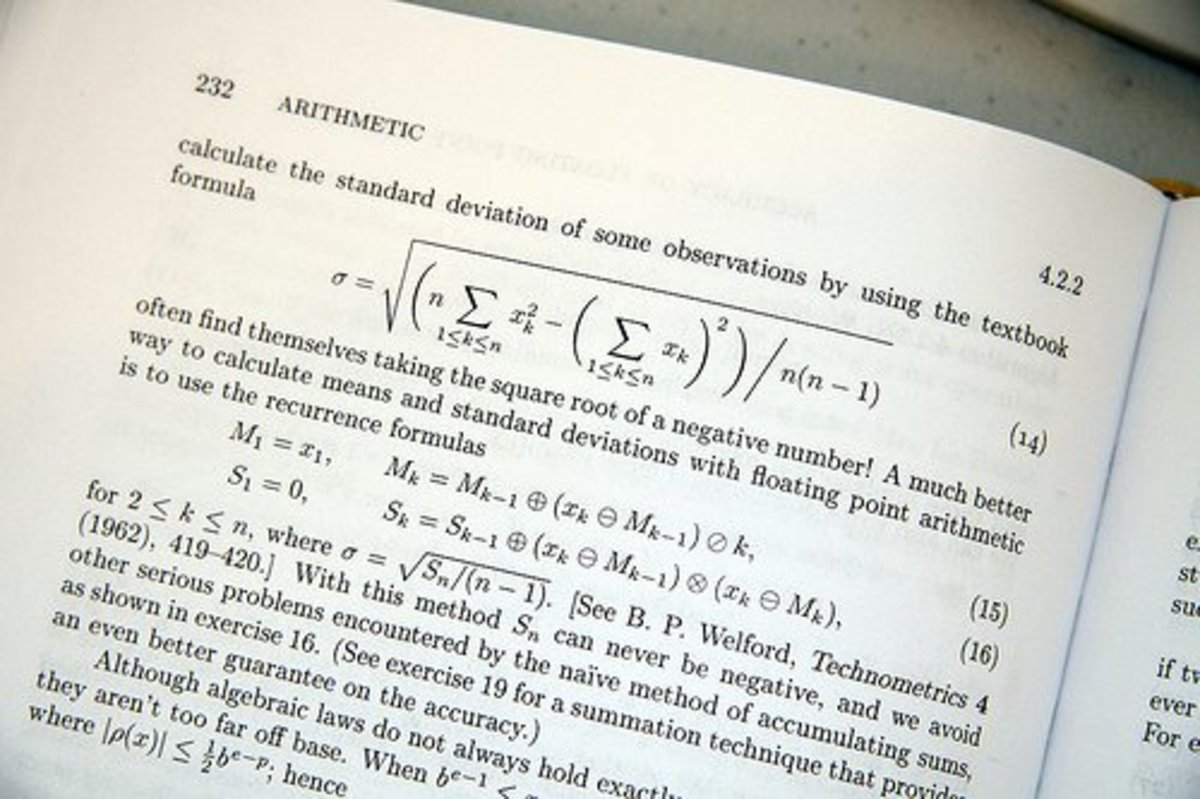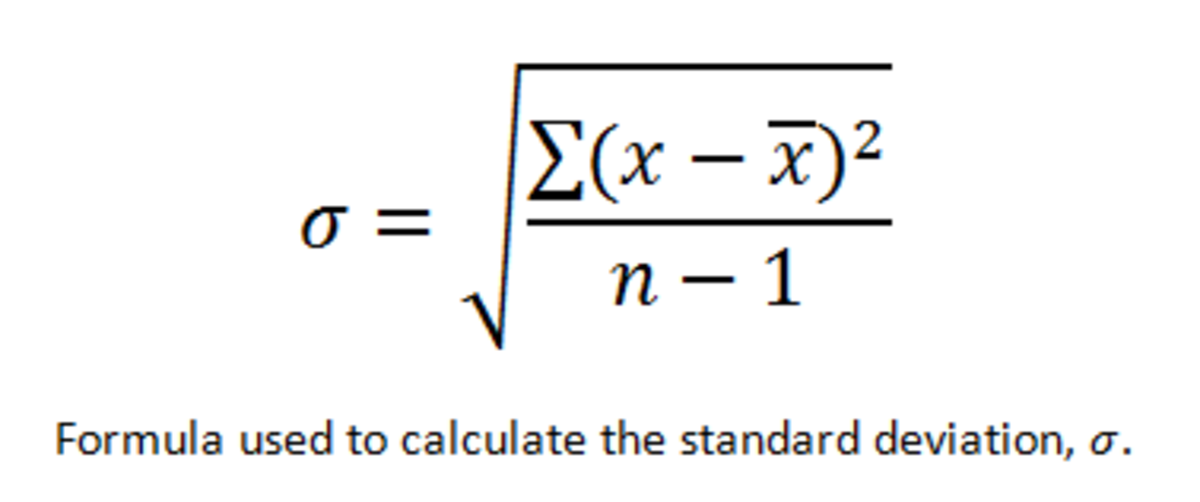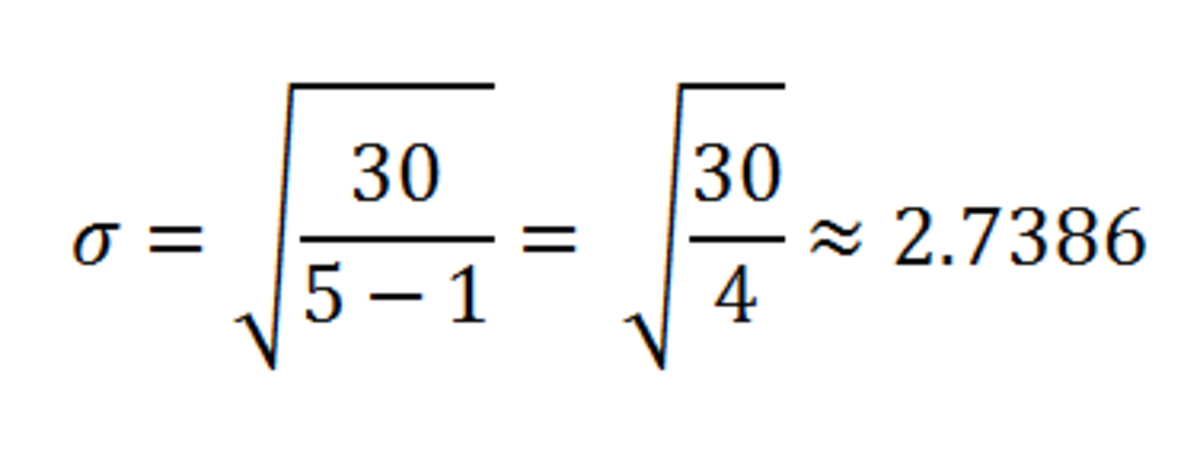Updated date:

# Standard Deviation and Variance By Hand: Steps to Calculate

## Let's Define Standard Deviation

The standard deviation of a population (by population, I mean a set of numbers), provides information of how much variation exists from the average value or the mean.

So, the standard deviation tells us about the variation in some population or set of data.

The standard deviation of a population is most-often represented by the lower-case Greek symbol sigma, σ.Learning how to calculate standard deviation is much less complicated than you think.chadmiller, CC-BY SA, via flickr.

## Equation for Standard DeviationWe will be using this to calculate the standard deviation of a population.Mariexotoni

## What does the standard deviation formula mean?

In order to calculate the standard deviation of some population, we must understand the notation in the equation.

Let's have a look at the chart below:

## Standard Deviation Formula Notation:

SymbolMeaning

Σ (sigma)

the sum across values

x

a number in the population

the average of the population

n

amount of data in population

## How to Calculate the Standard Deviation:

Overview of Steps

1. Calculate the mean of all numbers

2. Calculate the difference between each value and the mean

3. Square numbers from step 2

4. Take sum of squares

5. Solve for SD formula

## Standard Deviation Example

Step 1: Find the mean - To find the average of a population, you must first take the sum of the numbers and divide your result by the amount of numbers you added up (the amount of numbers in your population.

Example)

Here's a set of values: {12, 6, 5, 8, 9}

Find the sum of the set by adding all the values together: (12 + 6 + 5 + 8 + 9) =40

Notice the number of values in the population: n = 5

Take the sum of the values and divide by the numbers in the set: (40 / 5) = 8

Average = 8

Step 2: Difference between each value and the mean - In this step, you subtract the average found in step 1 from every value in your population. Some numbers may be negative.

Example)

Here's our set: {12, 6, 5, 8, 9}

Average: 8

Difference between values and mean:

(12 - 8) = 4

(6 - 8) = -2

(5 - 8) = -3

(8 - 8) = 0

(9 - 8) = 1

Step 3: Take the squares- Now, take the squares of all the values found in step 2.

Example)

Values found in step 2: (4, -2, -3, 0, 1)

Square all values: [42, (-2)2, (-3)2, 02, 12] = (16, 4, 9, 0, 1)

Step 4: Take sum of the squares - In this step, we take all the values we squared from step 3 and add them together.

Example)

Values from step 3: (16, 4, 9, 0, 1)

Sum the squares: (16 + 4 + 9 + 0 + 1 ) = 30The standard deviation of the example provided in the article is approximately 2.7386.Mariexotoni

Step 5: Solve the standard deviation formula - At this point, all the information needed to obtain the standard deviation has been found. Simply, we must plug in these values into the equation.

Example)

Sum of squares from step 3: 30

Number of values in set: n = 5

Standard deviation: sq. rt. ( 30/ (5-1))= sq. rt. (30/4) = 2.7386

## Final Quiz: Find the standard deviation

For each question, choose the best answer. The answer key is below.

1. Find the standard deviation of {6,12,9,5}
• 8.46
• 3.16
• 9.76

1. 3.16

## Standard Deviation Online Calculator

To make sure you've performed the standard deviation correctly, use the standard deviation calculator provided in the link below.

Standard Deviation Calculator

This is a good tool to use if you have a large set of numbers- for instance, if you have a population of 20 values, I recommend using a standard deviation calculator, or set it up to calculate the standard deviation in Excel or a program similar. If there's still any confusion the Khan Academy video at the side clearly demonstrates how to perform standard deviation- it's a useful tool from a trusted, well-established source.

Also, another great computational tool you can find at Wolfram Alpha's Site. You can calculate averages, standard deviations, variances and much more.

Point is- there is a plethora of resources available to you just by a simple Google search to find the standard deviation, if you are the type of person that needs to see the problem be walked through.

## Definition of Variance

Variance is a measure of how far a set of numbers is spread out, or how varied the set is! Once you find the standard deviation, you only have one more step to complete and you've found the variance! It's so easy and simple!

## How to find the variance

Step 6: Square the standard deviation- We find the variance by squaring the standard deviation.

Example)

Square the standard deviation: (2.7386)2= 7.50

Variance = 7.50

## Variance Online Calculator

Here is a calculator that calculates everything to do with standard deviation. The variance is the fourth one down. Here is the variance calculator.

Any questions? Leave them below.

ql on August 22, 2013:

Thanks a million!!!!!!!!!!!! it was so help ful

mariexotoni (author) on May 09, 2013:

thank you spond741- means a lot.

spond741 on May 09, 2013:

I like your blog. Keep up the good work.

http://communispond.com/

mariexotoni (author) on March 20, 2013:

welcome sarwar!! glad you think it's great!

sarwar on March 20, 2013:

this is really great!!!thank you.

mariexotoni (author) on December 22, 2012:

Thank you, kschimmel!

Kimberly Schimmel from North Carolina, USA on December 22, 2012:

Very nice hub. Good use of headings, graphics and text.